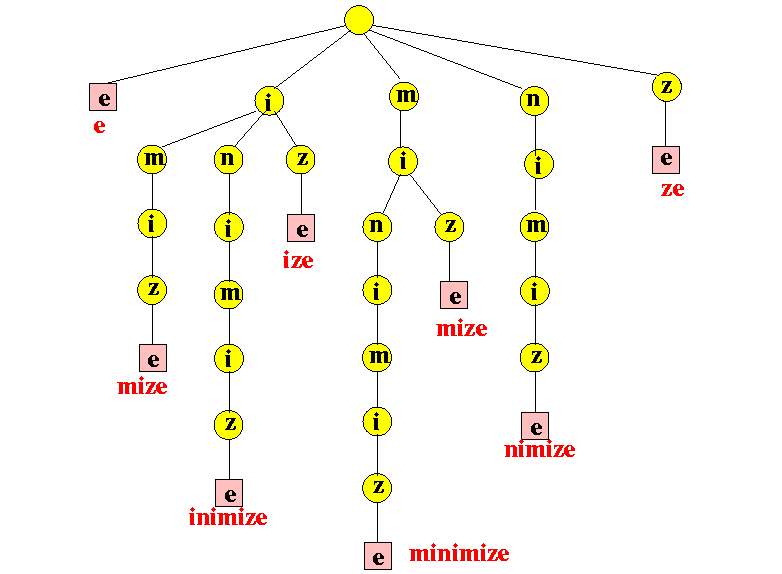# Pattern Searching using a Trie of all Suffixes

## Introduction

Trie is a special type of search tree data structure that is used as an efficient retrieval or searching data structure as searching time can be reduced to the optimal limit of pattern length. Here we will see one of the important implementations of trie for pattern searching.

But we will use standard trie of all suffixes to implement the pattern searching algorithm.

## Problem statement

You are given a text or string. You need to find all occurrences of given queries of patterns in the text.

Input
txt="minimize"
pattr="mi"

Output
Pattern found at position 0
Pattern found at position 4

Note: Please try to solve the problem first and then see the solution below.

## Approach

Step 1: building trie with all suffixes of the given text

• First, generate all suffixes of the given string
• Then, build the trie considering every suffix as individual words.

Step 2: pattern searching in trie

• Start from the root of the trie and the first character of the given pattern.
• If there is an edge from the root for the current character, follow the edge.
• Thus following the edges one by one for the current character if all characters of the given pattern are processed, the pattern is present in the text. We will store indexes of the suffixes starting at the current node.
• If there is not any edge for the current character of the pattern, then the pattern is not present in the given text.

## Dry run

Let the given string or text be “minimize”.

All possible suffixes of the given text :

1. minimize
2. inimize
3. nimize
4. imize
5. mize
6. ize
7. ze
8. esource

Output

## Complexity

Time complexity is O(n+k), where n is the length of the pattern and k is the number of occurrences of the pattern.

## FAQs

1. What is the Trie?
Trie is a special type of search tree data structure that is used as an efficient retrieval or searching data structure as searching time can be reduced to the optimal limit of pattern length.

2. What are some applications of the Trie data structure?
Trie is used in many string search-based applications such as autocomplete, prefix matching, text search.

## Key Takeaways

This article covered how to implement the pattern searching algorithm using the trie of suffixes.

Check out the CodeStudio library for getting a better hold of the data structures and algorithms.
Side by side, you can also practice a wide variety of coding questions commonly asked in interviews in CodeStudio. Along with coding questions, you can also find the interview experience of scholars working in renowned product-based companies here.

Happy learning!